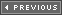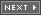### Real 2D Object-to-Object Collision Response (Advanced)

I put this section off a bit and moved it down here because I wanted you to really get a handle on momentum and collision and the mathematics needed to work with both. But like Dr. Brown said in Back to the Future, "Roads? Where we're going, we don't need roads…" Alas, object-to-object collisions with a fairly realistic collision response aren't the easiest thing in the world to figure out. The final results aren't bad, but coming up with them is no picnic. Anyway, let's get started.

Figure 13.33 depicts the general problem that we want to solve. There are two objects modeled by 2D circles or 3D spheres, and each has a mass and an initial trajectory. When they make contact we want to compute the final trajectory or velocity after the collision. We've touched on this already in the section "The Physics of Linear Momentum: Conservation and Transfer" when we came up with the following equations:

##### Figure 13.33. The central impact of two masses problem.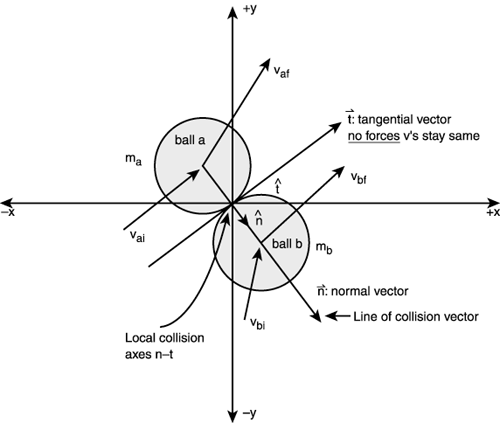Conservation of linear momentum:

```ma*vai + mb*vbi = ma*vaf + mb*vbf
```

Conservation of kinetic energy:

```1/2*ma*vai2 + 1/2*mb*vbi2 = 1/2*ma*vaf2 + 1/2*mb*vbf2
```

After combining them and solving for the final velocities, we get

```vaf = (2*mb*vbi + vai*(ma – mb))/(ma + mb)
vbf = (2*ma*vai - vbi*(ma – mb))/(ma + mb)
```

These equations are true for perfectly elastic collisions. However, there's a little problem—as they stand they are only 1-dimensional. What we need to do is come up with the 2D solution to the problem (something like a pool table) and this is a bit more complex. Let's start with what we know.

We know that each ball (2D representation) has some mass m; furthermore, the balls are made of the same material throughout, so the center of mass is at the center of the ball body. Next, we know that when real balls hit each other, the balls deform for a moment, some of the kinetic energy is converted to heat, and mechanical work to deform the balls, then the balls separate. This is called the impact event, which is shown in Figure 13.34.

##### Figure 13.34. The phases of the impact event.13.34.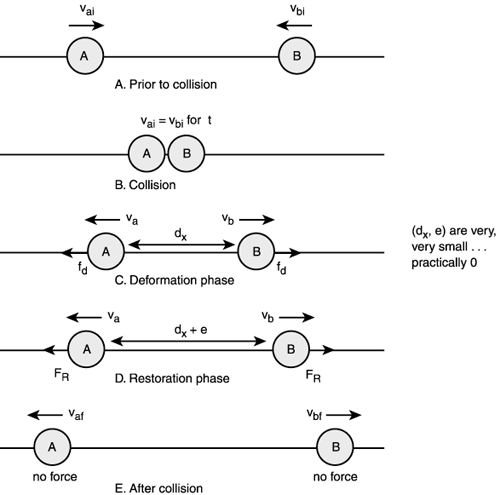The impact event consists of two separate phases. The first phase: Deformation, occurs when the balls make first contact and the balls move at the same velocity. At the end of the deformation phase the restoration phase begins and continues until the balls separate. The bottom line is that during the collision event a lot of really complex physics happen that we can't possibly model with a computer, so we have to make some assumptions about the collision. The cool thing is that even with the assumptions, when you see the simulation it will look pretty real! The assumptions are the following:

1. The time it takes for the collision event is very small; call it dt.

2. During the collision the positions of the balls do not change.

3. The velocity of the balls may change significantly.

4. There are no frictional forces acting during the collision.

Assumption 3 is the only one I need to clarify since I think the others are easy to swallow. For assumption 3 to be true, a force has to be applied during the collision that is almost instantaneous. This type of force is called an impulse force. This is the key to solving the problem. When the balls hit there will be a very large, short timed force that is created—an impulse. We can compute the impulses and from them come up with another equation to help solve the problem in 2D. The math is advanced and calculus based, so I will forgo it. The results are the generation of a coefficient that models all the physics during the impact event:

Equation 1: Coefficient of restitution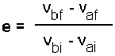Equation 1 is referred to by e and called the coefficient of restitution. It models the velocity before and after the collision and the loss of kinetic energy. If you set e=1 then the model is a perfectly elastic collision. On the other hand, e < 1 models a less than perfect collision and the velocity of each ball after the collision and the linear momentum will be less. Now where do you get e? e is something you set or look up. The interesting thing is that if you combine the equation for e along with the conservation of momentum equation:

```ma*vai + mb*vbi = ma*vaf + mb*vbf
```

you get the following results:

Equation 2: Final velocities

```vaf = ((e+1)*mb*vbi + vai*(ma – e*mb))/(ma + mb)
vbf = ((e+1)*ma*vai - vbi*(ma – e*mb))/(ma + mb)
```

Isn't that interesting? It's almost identical to the formulas we got when we combined the kinetic energy equations with the linear momentum equations. And in fact the assumption we made when we combined the kinetic energy equations with the linear momentum equations was that kinetic energy was conserved. If we assume that now then we set e=1 and we get

```vaf = ((1+1)*mb*vbi + vai*(ma – 1*mb))/(ma + mb)
vbf = ((1+1)*ma*vai - vbi*(ma – 1*mb))/(ma + mb)
```

or

```vaf = (2*mb*vbi + vai*(ma – mb))/(ma + mb)
vbf = (2*ma*vai - vbi*(ma – mb))/(ma + mb)
```

These indeed are the equations with both kinetic energy and linear momentum conserved! So it looks like we're on the right track. We have equations 1 and 2, so we should be able to solve the problem. But there's a catch; the equations are still in 1D, so we need to write them in 2D and then find a solution.

Referring back to Figure 13.33, you see there are two extra axes labeled—the n and t axes. The n axis is in the direction of the line of collision and the t axis or tangential axis is perpendicular to n. Assuming we have computed the vectors representing these axes (I'll show how a little later) then we can write some equations.

The first set of equations we're going to write relates the tangential component of the velocities before and after the collision. Since there are no frictional forces and no impulsive forces acting tangentially to the line of collision (trust me), the tangential linear momentum (and therefore the velocities) must be the same before and after, right? If there are no forces then this must be true, thus we can write

Equation 3: Relationship between initial and final tangential momentum/velocities

```ma*(vai)t = ma(vaf)t
mb*(vbi)t = mb(vbf)t
```

And if you wish you can combine them like this:

```ma*(vai)t + mb*(vbi)t = ma(vaf)t + mb(vbf)t
```

MATH

My notation is simple; (a,b) refers to the ball, (i,f) refers to initial or final, and (n,t) refers to the component along the n or t axes.

Since the masses are the same before and after the collision we can deduce that the velocities are the same by dividing the masses out:

Equation 4: Velocities after collision are equal in tangential direction

```(vai)t = (vaf)t
(vbi)t = (vbf)t
```

Cool. Now that we have half the problem solved we know the final velocities of the tangential components. Let's find the final velocities of the normal components, or the velocities in the line of collision n. We know that linear momentum is conserved always because there are no outside forces acting on the balls when they hit, so we can write:

Equation 5: Linear momentum is conserved in the n axis or line of collision

```ma*(vai)n + mb*(vbi)n = ma*(vaf)n + mb*(vbf)n
```

And we can also write e in terms of the n axis:

Equation 6: The coefficient of restitution in the n axis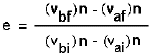Now let's take a look at what we have. If you look at equations 5 and 6, I have highlighted the variables that we don't have: (vaf)n, and (vbf)n. Just the normal components of the final velocity. Bingo! We have two equations and two unknowns, so we can solve for them. But we already have the answer! Equation 2 still holds for any particular axis, so I can rewrite it for the component along n:

Equation 7: Final velocities in the normal direction

```vaf = ((e+1)*mb*(vbi)n + (vai)n*(ma – e*mb))/(ma + mb)
vbf = ((e+1)*ma*(vai)n – (vbi)n*(ma – e*mb))/(ma + mb)
```

That's it!I l@ve RuBoard